Next: 5. Surface Rate Calculation Up: 4.10 Parallelization Previous: 4.10.2 Data Access

## 4.10.3 Benchmarks

To test the parallel efficiency, the surface evolution was calculated for a sphere expanding at constant speed. The calculation was performed using 1, 2, 4, 8, and 16 cores of AMD Opteron 8435 processors clocking at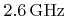. The corresponding average calculation times for a single time step and for different sphere diameters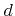(measured in grid spacings) are listed together with the parallel efficiency in Table 4.4.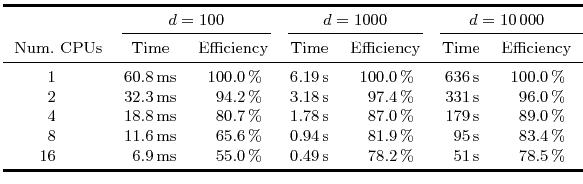According to Amdahl's law  the parallel efficiency decreases with the number of CPUs due to sequentially processed parts of the program. In case of 16 cores a parallel efficiency of approximately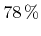could be achieved except for the smallest sphere diameter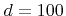. For smaller structures the overhead due to thread synchronization is more relevant, which results in a worse efficiency. Table 4.4 also shows the good scalability with surface size. If the diameter is multiplied by 10, the surface of the sphere is increased by a factor of 100, which is well reproduced by the listed runtimes.Next: 5. Surface Rate Calculation Up: 4.10 Parallelization Previous: 4.10.2 Data Access

Otmar Ertl: Numerical Methods for Topography Simulation